### Multi-coating design and pulse analysis

Most of the modern ultrafast laser systems include dispersive mirrors used for accurate phase control required for efficient pulse compression.

Dispersive mirrors compensate pulse broadening or deformation that ultrashort laser pulses experience as they propagating through optical dispersive media.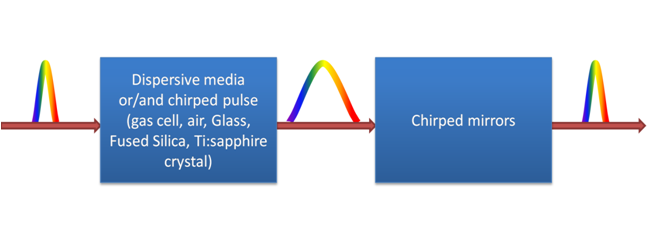Fig. 1. Schematic of pulse propagation through dispersive medium and pulse compression.

In the spectral domain, the field strength of the pulse can be written as:

$E_{input}(\omega)=\frac{E_0\tau_0}{2\sqrt{\ln 2}}\cdot\exp\left[-\frac{\tau_0}{8\ln 2}(\omega-\omega_0)^2\right],$

$\omega=\frac{2\pi c}{\lambda},$

$$E_0$$ is the pulse amplitude, $$\tau_0$$ is pulse duration, $$\omega_0$$ is a carrier frequency.

Propagating a distance $$z$$ through a dispersive medium with refractive index $$n(\omega)$$, the pulse accumulates a phase $$\varphi:$$

$\varphi(\omega,z)=\frac{\omega n(\omega)}{c}z.$

Derivatives of the phase, group delay (GD) and group delay dispersion (GDD), can be calculated using Material dispersion option.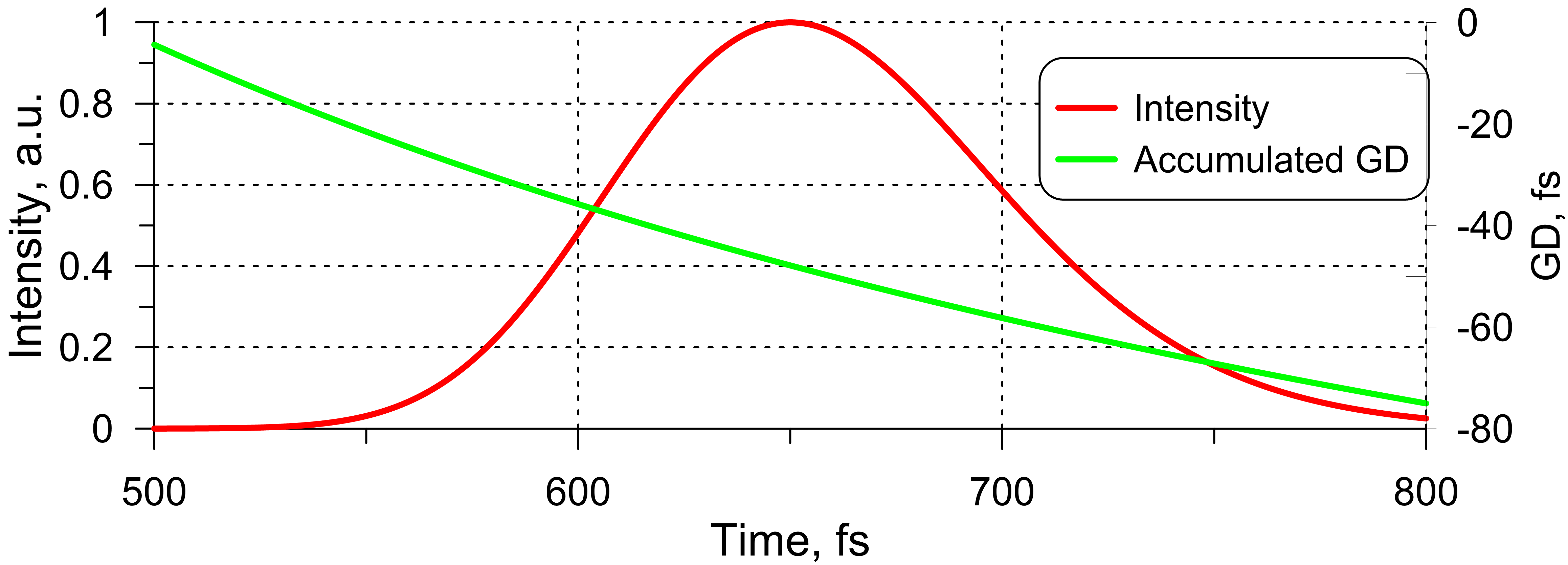Fig. 2. Input intensity and accumulated group delay (GD) of a pulse in spectral domain.

In the time domain, the pulse can be described as:

$E_{input}(t,0)=E_0\cdot\exp\left[-2\ln 2 \cdot\frac{t^2}{\tau_0^2}\right]\cdot\exp[i\omega_0 t]$

Phase derivatives, GD, GDD, and TOD (third order derivative), affect the temporal shape considerably (Fig. 3).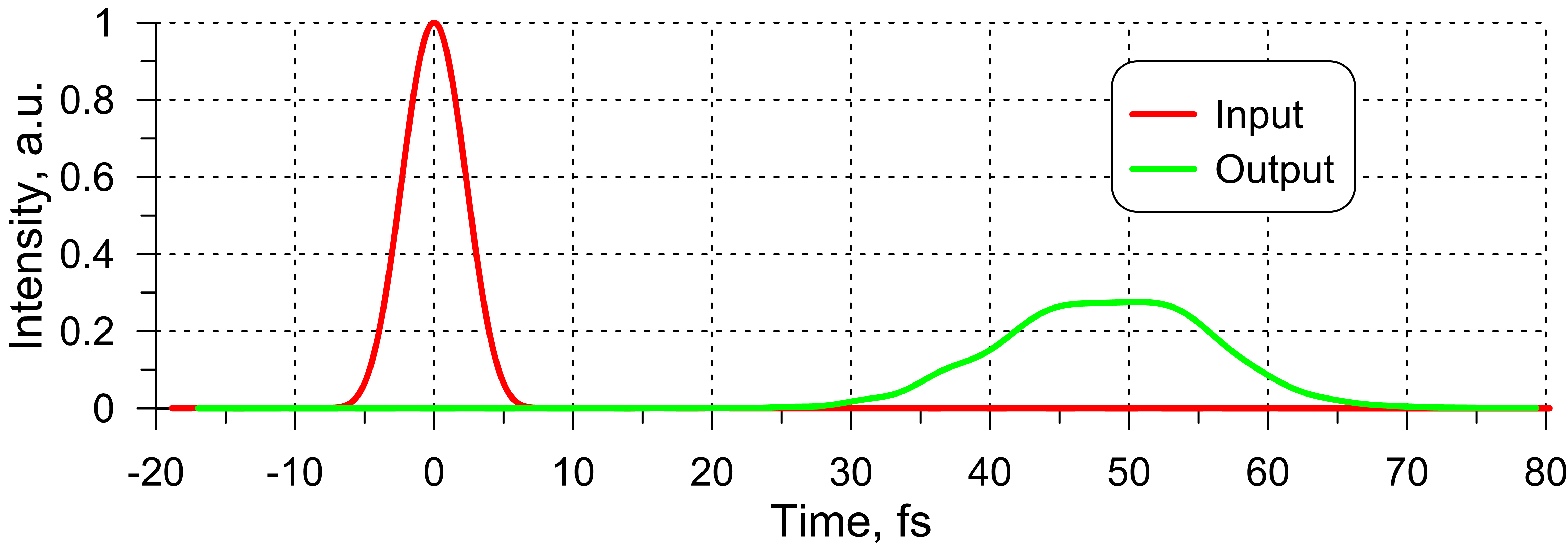Fig. 3. Input pulse envelope and output pulse after propagating through the medium with GD shown in Fig. 1.

In the simplest cases, when GDD is a constant, it influences the pulse amplitude and pulse duration. Constant GD effects the temporal position of the envelope only.

In more complicated cases, if GD is frequency-dependent or if GDD has oscillations, it may cause satellite pulses, deformation or even distortion of pulses.

Effect of +5 fs2 (Fig. 4) and -5 fs2 (Fig. 5) GDD on electric field distribution of the pulse. The bandwidth of the pulse is 500-800 nm, central wavelength 650 nm, pulse duration is 2.3 fs.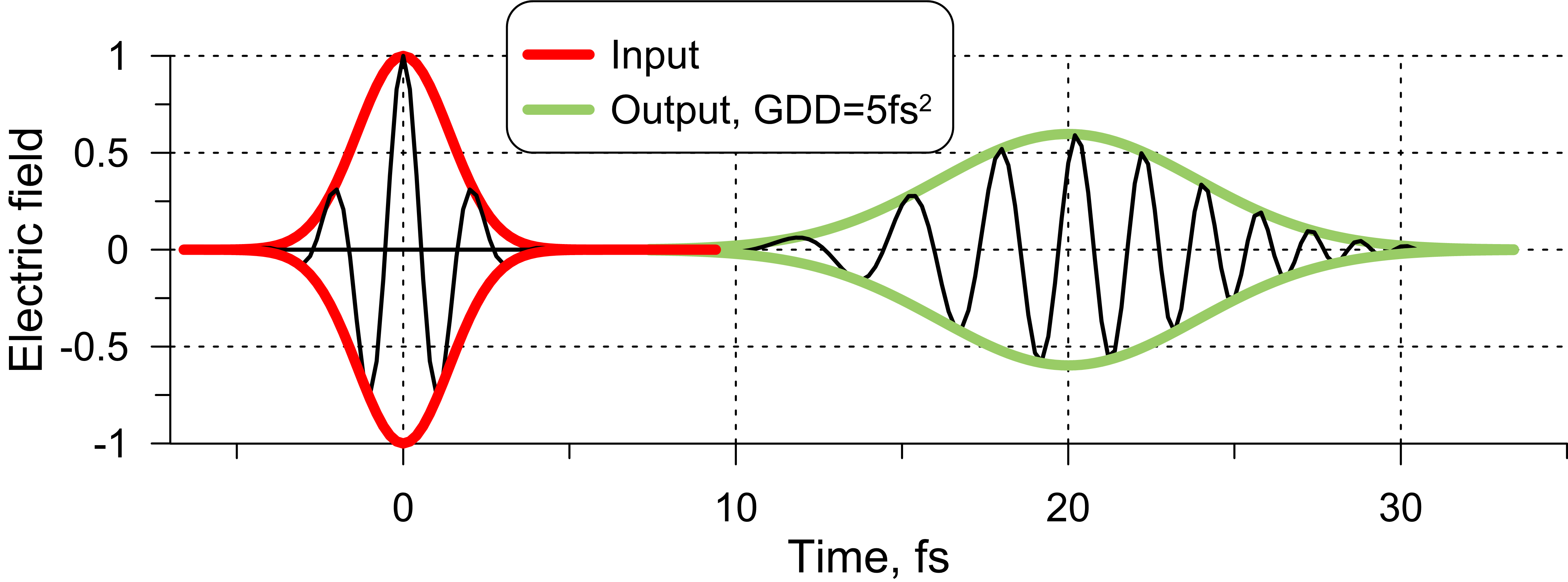Fig. 4. Electric field of a 2.3-fs pulse before and after propagating through a dispersive medium and accumulating +5 fs2 GDD.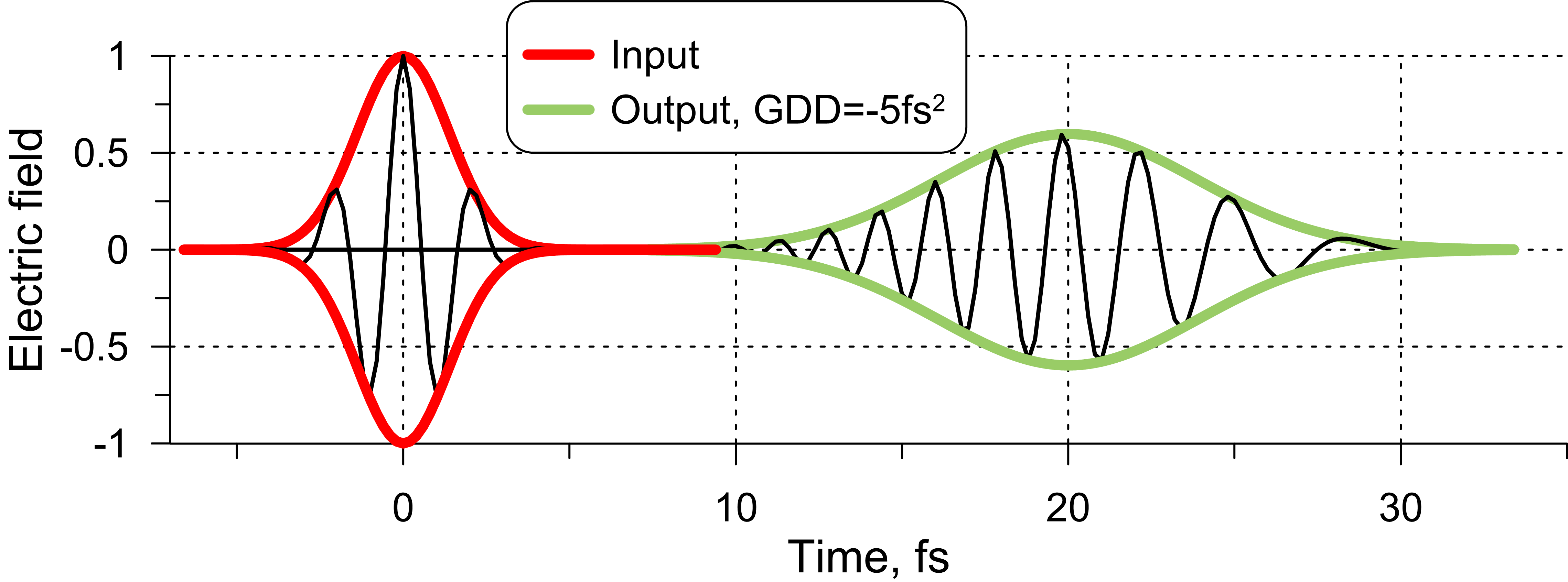Fig. 5. Electric field of a 2.3-fs pulse before and after propagating through a dispersive medium and accumulating -5 fs2 GDD.

In more complicated cases, GD and GDD can be dependent on spectral parameter (for example, Fig.6). This can lead to broadening and deformation of the pulse (Fig. 7).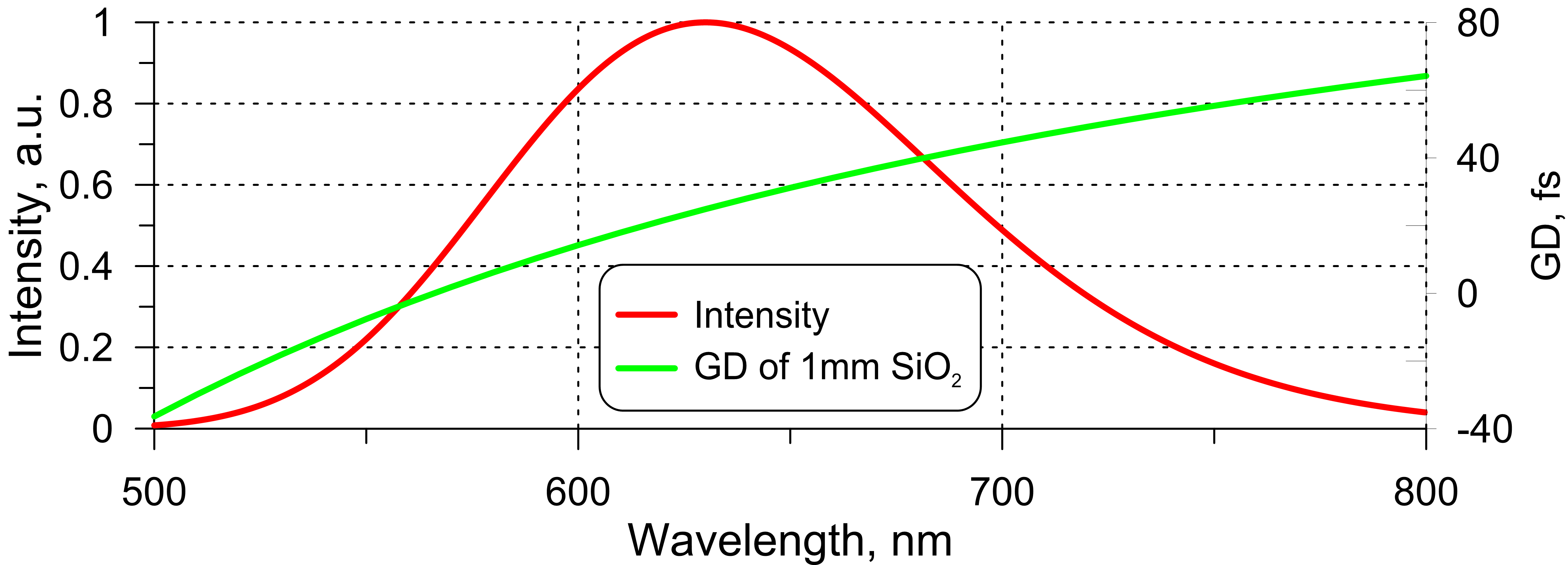Fig. 6. Input pulse in spectral domain and GD accumulated in 1mm SiO2.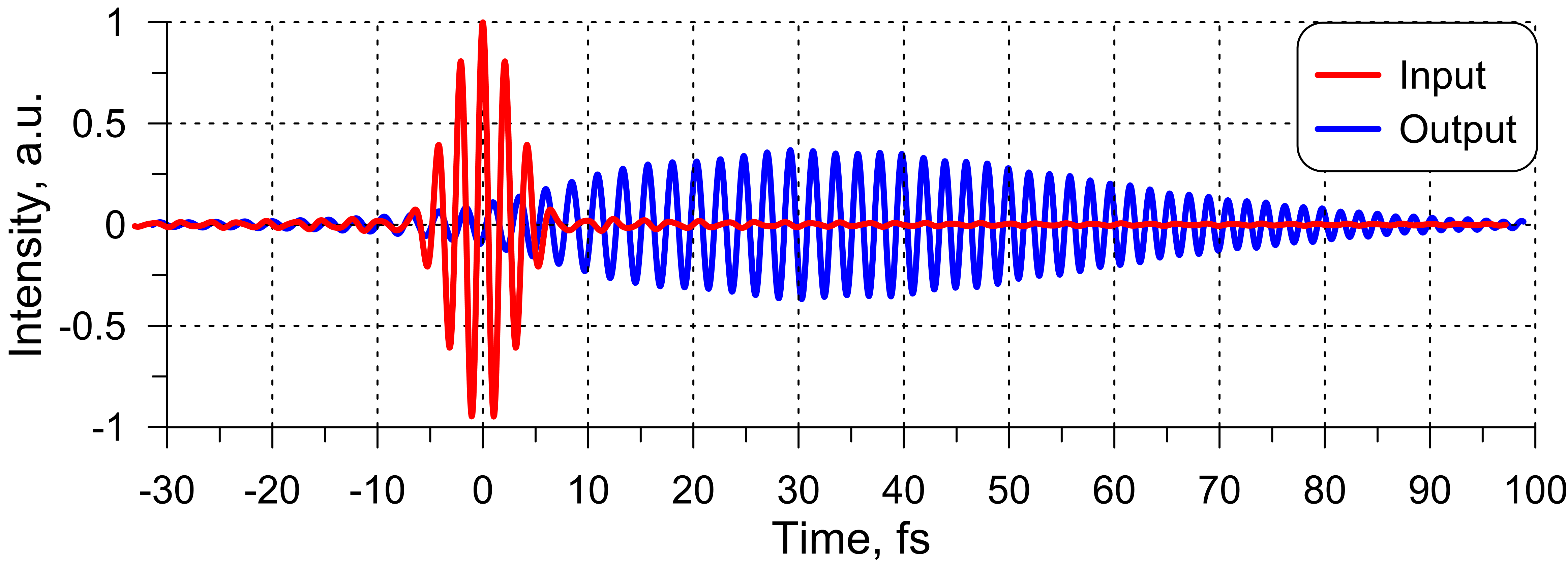Fig. 7. Input pulse in time domain and output pulse after propagating through a 1mm thick SiO2 medium.

### Broadband dispersive mirror: classical design

OptiLayer allows you to design dispersive mirrors and high-dispersive mirrors using needle optimization, gradual evolution, deep search versions of the needle optimization/gradual evolution, and floating constants approaches.

Example: dispersive mirror operating in the broadband spectral range from 500 to 800 nm and exhibiting GDD of -40 fs2. Reflectance exceeds 99.9% in the entire working spectral range. A 52-layer design solution can be easily obtained using Gradual Evolution with Deep Search. Pulse analysis option allows you to evaluate pulse intensity after interacting with the mirrors.Fig. 8. GDD of the 52-layer dispersive mirror.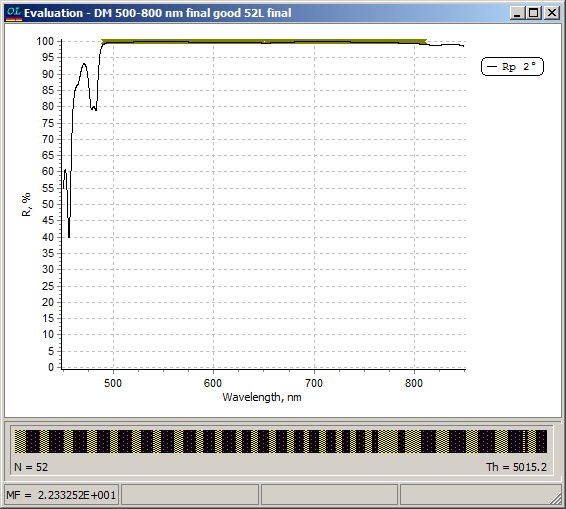Fig. 9. Reflectance of the 52-layer dispersive mirror.

GDD exhibits oscillations of about 40 fs2 amplitude. Nevertheless, pulse analysis shows that pulse duration does not change in the case of one bounce (Fig. 10)  and even for four bounces (Fig. 11). Reflectance drops by approximately 2% after four bounces (Fig. 11).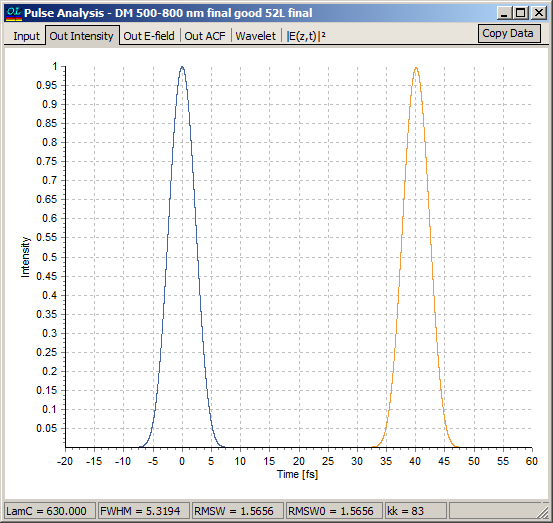Fig. 10. Input pulse intensity (blue) and pulse intensity after compression with the help of the 52-layer dispersive mirror (orange). One bounce.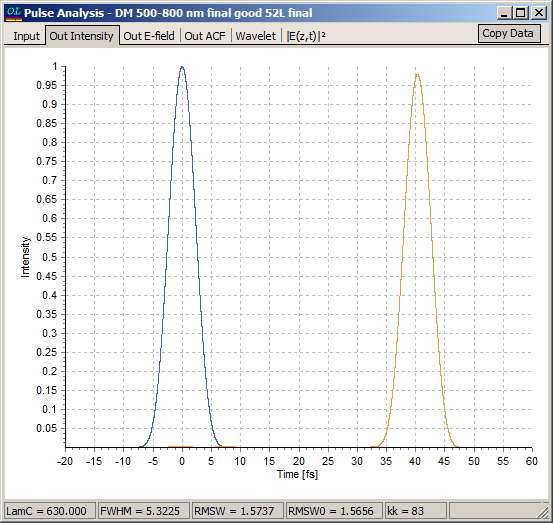Fig. 11. Input pulse intensity (blue) and pulse intensity after compression with the help of the 52-layer dispersive mirror (orange). Four bounces.

### Complementary pairs of dispersive mirrors

When the pulse bandwidth is getting broader, it is more and more difficult to suppress oscillations in GDD keeping up the highest reflectance. It is mathematically proved that oscillations of GDD are inevitable. It means that it is required to find a way to suppress them or to reduce their effect on the output pulse characteristics. In challenging cases, complementary pair of mirrors can be designed.

For dispersive mirror pair reflectance and GDD can be defined as:

$R_\Sigma=\sqrt{R_1\cdot R_2},\;\; GDD_\Sigma=\frac{GDD_1+GDD_2}{2}$

• It is possible to achieve suppression of GDD oscillations if GDDs for each mirror are almost in anti-phase;
• Production is extremely challenging since it is necessary to deposit precisely matching pair with extremely high accuracy;
• Special procedures for reducing sensitivity of the designs to the production errors, i.e., in order to find more robust design.

Example. Dispersive mirror operates in the spectral range from 500 to 1000 nm (one octave), GDD=-50 fs2, reflectance exceeds 99% in the entire spectral range.

First, it is recommended to design a "classical" mirror, oscillations of high amplitudes are possible (see, for example, Fig. 12). These oscillations reach 450-500 fs2. Such oscillations lead to decreasing pulse intensity (Fig. 13) that can be easily checked using pulse analysis option.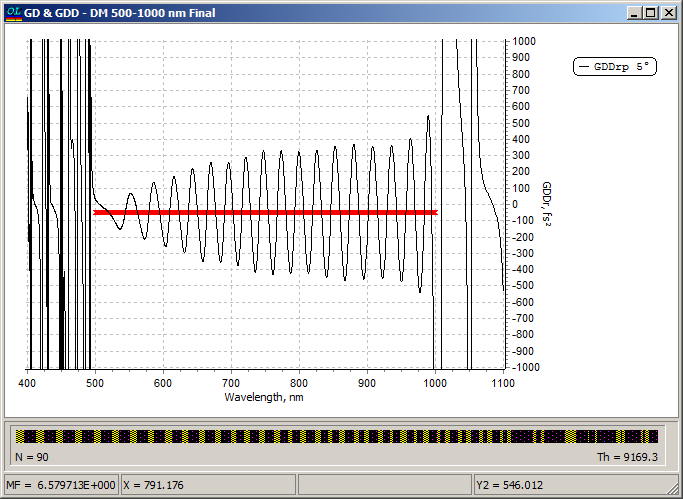Fig. 12. GDD of a 90-layer one-octave dispersive mirror.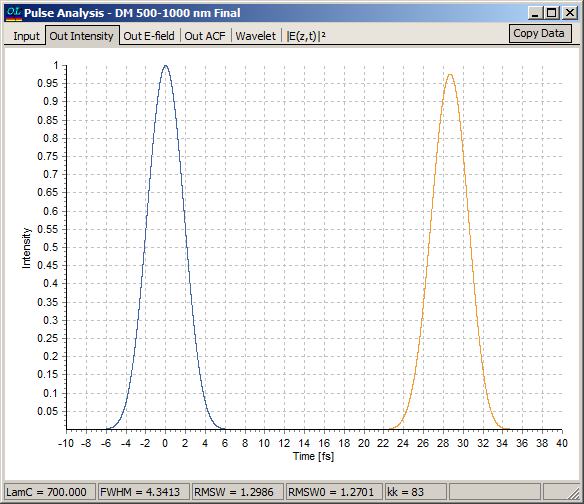Fig. 13. Input pulse (blue) and the pulse accumulated +50 fs2 GDD and reflected from the 90-layer one-octave mirror (orange). Decrease of the peak intensity is clearly observed.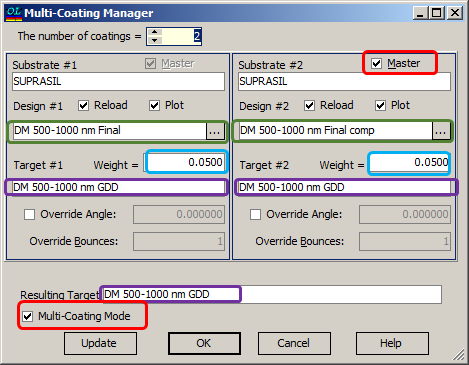Fig. 14. Specification of parameters for synthesis of the complementary pair operating in the range 500-1000 nm. The 90-layer dispersive mirror (Fig. 12) is specified in Design #1 field.

Second, in order to design a complementary pair you need to activate Multi-Coating Manager first: Data --> Multi-coating.

Multi-coating Mode checkbox must be checked. Master checkbox activates Complementary pair approach (Fig. 14).

In Design #1 and Design #2 fields, two dispersive mirrors designs should be specified as starting designs. One of them can be a classical design (Fig. 12) and the second one can be a design with slightly shifted optical layer thicknesses.

In Resulting Target field, a target for the complementary pair is to be specified.

In Target #1 and Target #2 fields, targets for each separate mirror should be specified. These targets can be identical or they can be different.

Typical setting of The number of coatings field is 2 (two mirrors). OptiLayer enables to specify up to 10 different coatings to design extremely complex dispersive mirror compressors.

After pressing Update or OK button, you can start Refinement or Needle optimization algorithms.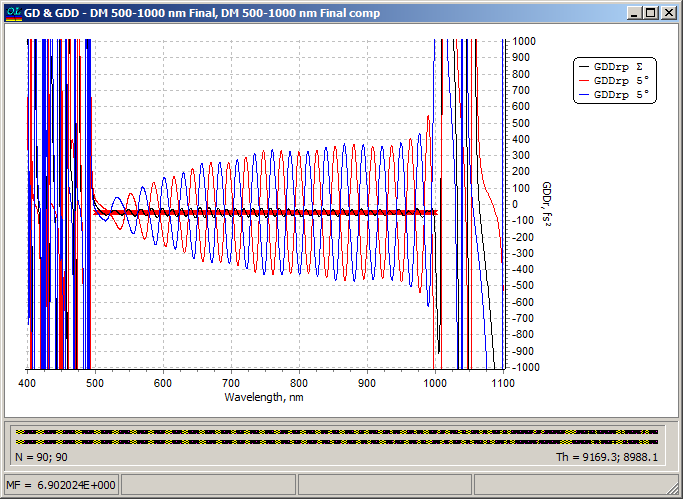Fig. 15. GDD of two separate mirrors of the complementary pair and GDD of the pair. GDD oscillations of separate mirrors compensate each other and resulting GDD oscillations are very low.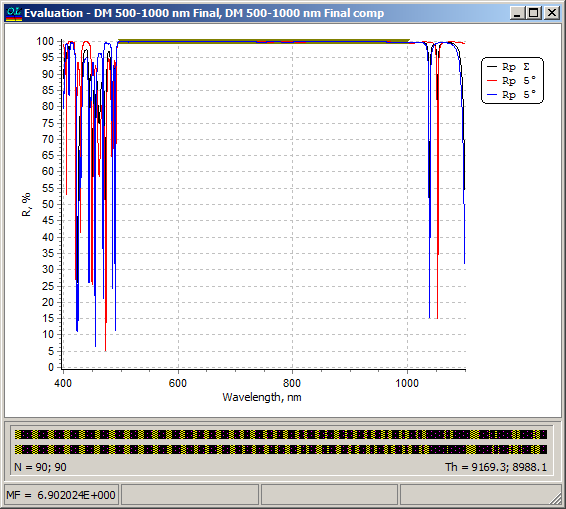Fig. 16. Reflectance of two separate mirrors of the complementary pair and resulted reflectance of the pair.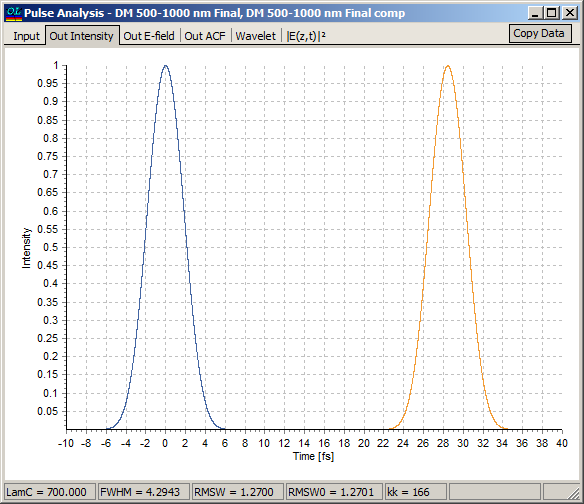Fig. 17. Input pulse (blue) and the pulse accumulated +50 fs2 GDD and reflected from the complementary pair (orange).

Pulse analysis helps you to evaluate the quality of the designed mirrors.

Pulse analysis of the complementary pair (Fig. 17) shows that there is no decrease of pulse intensity as in Fig. 13.

### Double angle approach

Another way to solve challenging design problems is double angle approach. There are several advantages of this approach in comparison with the complementary pair approach.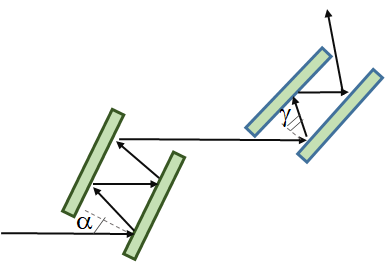Fig. 18. Schematic of a double angle configuration.

• Double angle approach allows you to design feasible broadband dispersive mirrors, which can be produced in a single deposition run. Inherent oscillations of the GDD are suppressed by using the mirrors at two different angles of incidence (Fig.  18).
• Since the mirrors designed at two different incidence angles are produced in a single coating run, their overall dispersion is more robust to errors in layer thicknesses than in the complementary mirror pairs produced in two different runs.

Example. Dispersive mirror operates in the spectral range from 670 to 910 nm, GDD=-150 fs2, reflectance exceeds 99% in the entire spectral range.

As in the case of complementary pair approach, it is recommended to design a "classical" mirror, oscillations of high amplitudes are allowed (see, for example, Fig. 19).

These oscillations reach 100 fs2. Such oscillations may lead to decreasing pulse intensity that can be easily checked using pulse analysis option.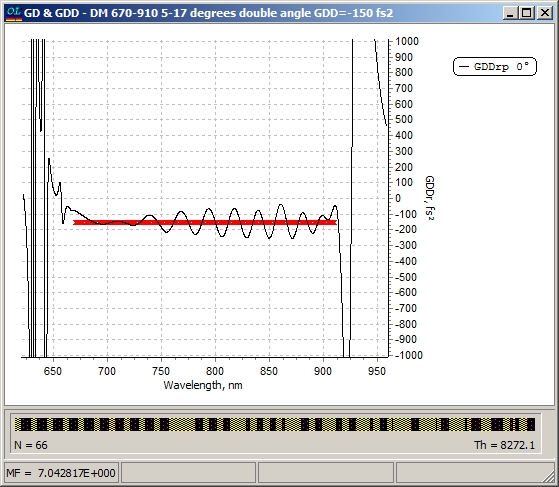Fig. 19. GDD of a 66-layer dispersive mirror.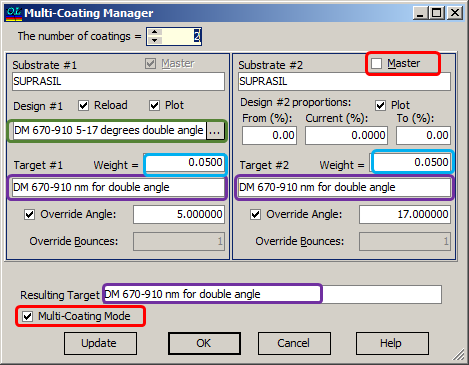Fig. 20. Specification of parameters for synthesis of the double-angle mirrors operating in the range 670-910 nm. The 66-layer dispersive mirror (Fig. 19) is specified in Design #1 field.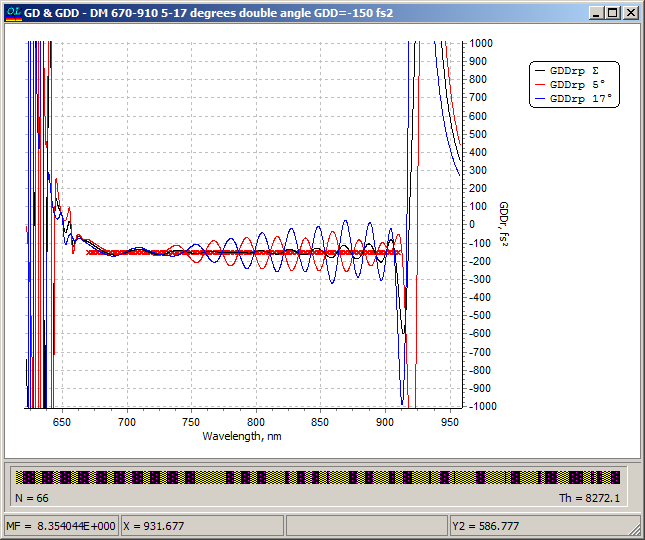Fig. 21. GDD of the optimized 66-layer dispersive mirror at 5°, 17°, and resulting GDD of two mirrors (configuration is schematically shown in Fig. 18). GDD oscillations of the mirrors at different AOI compensate each other and resulting GDD oscillations are very low.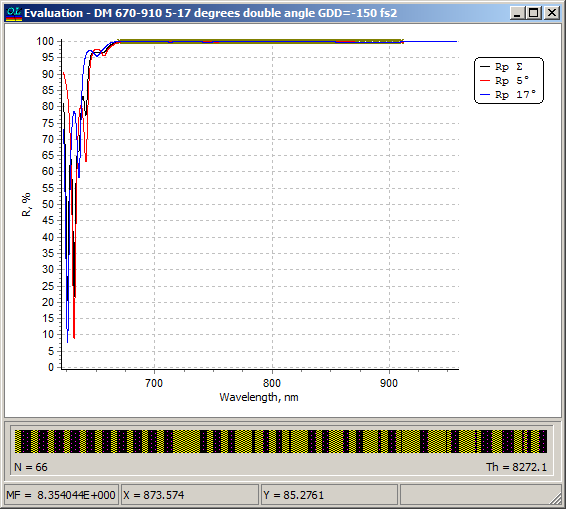Fig. 22. Reflectance of the optimized 66-layer dispersive mirror at 5°, 17°, and resulting reflectance of two mirrors configured as it is shown in Fig. 18.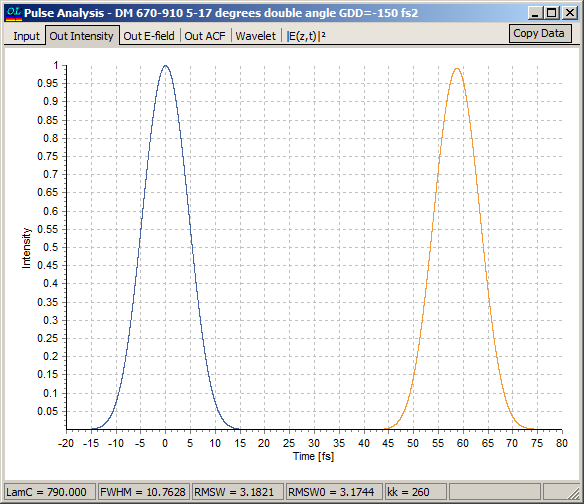Fig. 23. Input pulse (blue) and the pulse accumulated +150 fs2 GDD and reflected four times from the 66-layer dispersive mirrors in the configuration schematically shown in Fig. 18 (orange).

### Pulse analysis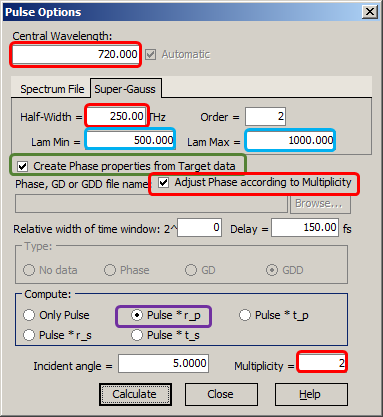Fig. 24. Settings for pulse analysis: central wavelength, pulse width, bandwidth.

In ultrafast optics it is often important to study how temporal shape of reflected pulse looks like.

The pulse analysis option is extremely important for designing dispersive or chirped mirrors.

Pulse options settings (Fig. 24) are available through

Analysis --> Pulse Analysis

OptiLayer shows input pulse and GD/GDD to be compensated as, for example, in Fig. 2.

OptiLayer represents the intensities of Fourier-limited and output pulses in time domain, therefore  it is very convenient to estimate the quality of compression and expected distortions, as in Figs. 10, 11, 13, 17, 23.

For example, in this case the compression is not good, you will see satellites after the pulse. The problem appeared due to deviations of average GDD level of the designed mirror from the target values.

OptiLayer calcualated and represents approximating time behavior of the electric field in a pulse (both Fourier-limited and output pulse).

Instead of a model Gaussian pulse, you can specify your spectrum file.

It is important that pulses are simulated taking the multiple bounces (multiplicity) into account.

### Easy to startOptiLayer provides user-friendly interface and a variety of examples allowing even a beginner to effectively start to design and characterize optical coatings.        Read more...

### Docs / SupportComprehensive manual in PDF format and e-mail support help you at each step of your work with OptiLayer.If you are already an experienced user, OptiLayer gives your almost unlimited opportunities in solving all problems arising in design-production chain. Visit our publications page and challenge page.